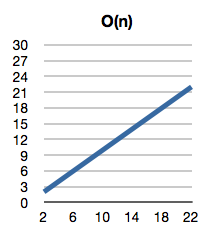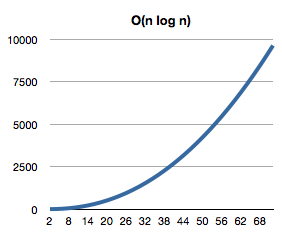Trying to understand how an algorithm works? Are people trying to tell you how effective it is? They’re probably using Big O Notation.

Big O Notation (also known as Big Oh notation, Landau notation, Bachmann–Landau notation, and asymptotic notation) is used to help developers and engineers measure the speed or memory an algorithm will take. It generally measures the best, worst, and/or average case scenario. This is a short guide, for more information look at this recourse where I got a lot of this information.

Big O Notation always contains a formula inside of parenthesis that indicate the information you will need to know. You will often see an n in the formula, this n is indicative of the number of elements in an array. You may also see logarithms, exponents, or other mathematical values or computations. Here is a list of some common O notations and what they mean.

O(1) O(1), O(101), or any other number describes an algorithm that will always finish in the same time. It will take exactly as long or as many recourses to make its calculation no matter how long or short an array of data is. If you were to view this visually, you would see a flat line on a graph.O(n) O(n) describes a linear relationship between the number of data elements and the amount of time or memory an algorithm will take. In other words, an array with 100 elements will take 10 times as long as an array with just 10 elements. Visually you would see a straight line increasing upward at the same rate that is it progressing forward.

O(n2) O(n2) describes an exponential relationship between the number of elements in an array and the amount of time it will take to complete. On a graph this would be exponential line where the line starts at a slight incline and progresses to a near vertical slop. (We like to avoid these kind of algorithms if at all possible, since they are memory hogs.)

O(n log n)O(n log n) indicates that an algorithm will take the number of elements times the logarithm of the number of elements. If you were to look at a graph of this, it wouldn’t be linear and it would be exponential, it would be somewhere in between. You would see a slop that started out climbing at a quick pace and then slowed it’s progress to appear more linear.

Conclusion Obviously, there are many permutations of this practice (as there are an infinite number of ways to write an equation) but you get the idea. Feel free to ask any questions? I hope I’ll have the answer. ;)

Great post! But maybe if you add examples to each case, e.g. O(n log n) is for merge sort, etc. Thanks!
bobo on 2013-09-08 16:55:02.0